Volume 1, Number 2, pp. 1-5, 1999.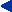Home  Current Issue  Table of Contents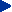Comments on "Review of Methods for Solving the EEG Inverse Problem" by R. D. Pascual-Marqui

Functional Brain Mapping Lab., Department of Neurology, University Hospital Geneva (HCUG)
rue Micheli-du-crest 24, 1211, Geneva 14, Switzerland

Comments on "Review of methods for solving the EEG inverse problem" by R.D. Pascual-Marqui .

First of all we would like to state some opinions or facts related to the general philosophy of linear distributed inverse solutions. For proofs, clarifications or more extensive discussions we refer the interested reader to ( , , , ).

a) The goal of a distributed inverse solution is to estimate the position, strength and extent of multiple simultaneously active sources. If only a few ideal point sources are known to be active a multidipole localization method should be preferred.

b) The properties of a linear distributed solution to localize multiple active sources can only be inferred from its properties to localize single sources if :

• The superposition principle holds (as is the case for the resolution kernels or the spread functions but no for the dipole localization error)
• Adequate localization is understood not only in terms of an adequate estimate of the positions of the sources but also in terms of a correct estimation of the source strength.

c) The bioelectromagnetic inverse problem aims to estimate the current source density within the brain (or heart), i.e., a vector field. The magnitude submitted to analysis in tables and figures in ,  is the modulus of the estimated vector. The modulus of the vector field, is non linearly related to the components of the vector. The superposition principle cannot be applied to a non linear relationship. For instances, given two single dipoles d1 and d2, the dipole localization error (E) as used in  and  in general will satisfy that E(d1+d2)1E(d1) + E(d2) and thus is not additive (a necessary condition for superposition).

These few remarks will help us to clarify the points on which we disagree with Pascual-Marqui that are:

1) "An exhaustive study of all possible spread functions constitutes a complete characterization of an inverse solution".

The study of all possible spread functions is equivalent to the analysis of all the resolution kernels , . However, the analysis presented by Pascual-Marqui in  and  to evaluate the solutions is not really using the spread functions but a measure derived from them: The dipole localization error. As we state in c), the superposition principle does not hold for this magnitude. Moreover, the dipole localization error is not even defined in  or  for the addition (combination) of two single sources. Furthermore, an exhaustive study of the spread functions implies not only the analysis of the deviation of the position of the maxima but should also include the analysis of the estimated amplitude (estimated source strength) which is absent in  and  (see b). Only by taking into account the source strength it is possible to understand why a solution could provide adequate maps for single sources while totally failing for some linear combinations of the same sources .

2) About the "futility of trying to design near ideal averaging kernels"

The author concludes from the harmonic character of the lead fields the futility of optimizing the resolution kernels (RK). The impossibility of obtaining ideal RK for underdetermined inverse problems (with or without harmonic lead fields) has been extensively discussed in the literature (, , ). In , it is explicitly mentioned that "since the RK are constrained to the space spanned by the rows of the lead field, they are intrinsically a determined by the physical laws relating sources to measurements " and thus that "resolution kernels cannot be manipulated at will". In  and  the same aspect is again extensively discussed and the impossibility of obtaining delta like resolution kernels for non boundary points is also illustrated by simulations. Despite these facts we insist in the rationality of using this alternative strategy for the design of a distributed inverse solution (see a), i.e., to demand minimum of interference from possible simultaneously active far away points in the estimator of the activity at the target point. This can be also concluded from the works presented by A. Dale and F. Babiloni in previous Human Brain Mapping meetings. In addition, Pascual-Marqui fails to note that optimizing the resolution kernels without the unimodularity constraint (as in the WROP method) is mathematically equivalent to optimize the point spread functions (Appendix I). Then, following Pascual-Marqui's reasoning we have to conclude that there is no sense in optimizing the spread functions neither, what obviously contradicts his claims that optimality of the spread functions (in the sense of low dipole localization error) is a "minimum necessary condition".

We are pleased to see that in this paper , the author coincides with us (, , , , ) in that the averaging kernels contain information about how the estimators at some given point is influenced by all possible active sources. Based on this interpretation we have emphatically proposed the use of the resolution kernels and measures derived from them to evaluate the performance of distributed linear inverse solutions (, , , ), in contrast to the use of measures derived only from the point spread functions as used by Pascual-Marqui , .

3) About the comments on the WROP method:

It is not true that we "omitted an explicit equation of the inverse solution for the case of an unknown vector field". The explicit equation to compute each row of the WROP inverse (for arbitrary selected weights) is equation (14) in . In addition an explicit expression to compute the weights is included. These equations are completely valid for the magnetic case once the lead field is properly transformed to consider only a tangential vector field. It is well known in mathematics that any set of underdetermined homogeneous linear constraints can always be incorporated as a transformation of both, the lead field and the inverse of the transformed lead field (Appendix II). This approach allows to write compact equations, as we did in , valid for any type of volume conductor model and for both the magnetic and the electric case. Thus, all the results described in  take this aspect into consideration. Unfortunately, the 3D plot used in  does not allow to say whether or not the radial component is zero.

Finally, the author fails to realize that the WROP method is not a particular inverse solution but an strategy to encompass in an unified formulation a family of linear distributed solutions that includes as particular cases the minimum norm solution, all weighted minimum norms, and many other complex metrics that, to our knowledge, nobody has explored so far. Obviously, to infer the localization properties of all the solutions that belongs to this family from some simulations performed with a particular example of the weights is impossible. For instance, two members of the WROP family: the minimum norm and the radially weighted minimum norm " differ completely in their capabilities to reconstruct single sources at different depths.

One of our main concerns is to convince the reader why the dipole localization error of single sources alone does not allow to predict the capabilities of a distributed inverse solution to retrieve more complex source configurations. This can be illustrated by simple simulations (a theoretical example is considered afterwards) using a few simultaneously active sources. Lets consider for example three single sources d1, d2 and d3 with very low dipole localization errors. The answer to the question: How does the inverse behave when the three sources are simultaneously active, depends also upon the strength estimated for each source. Only when the estimated strengths for the three sources is comparable we will observe in the reconstructed instantaneous map the presence of the three sources. However, the estimated strengths degrades with the eccentricity of the sources and thus, sources of actual similar strength at different eccentricities will be hardly detected in the reconstructed maps. The analysis of the errors of the solutions in the estimation of the single source strengths is absent in  and , decreasing dramatically the value of the comparisons presented.

The 3D bioelectromagnetic inverse problem is extremely underdetermined because we are trying to explain the sources of the measured data (generators) that have a huge number of degrees of freedom (at least equal to the number of solution points, Np) with just a few constraints (the number of sensors, Ns) (these two dimensions are exactly the dimensions of the inverse matrix , a few columns and a lot of rows). Obviously with only Ns columns we cannot describe all possible changes that can arise in an Np dimensional space. Lets consider a theoretical example that might be helpful to understand which are necessary conditions to design adequate inverse solutions to the problem. Let be G (for good) and B (for bad) two inverse matrices such that G retrieve almost perfectly all single sources while B yields to poor dipole localization errors. If the actual generators are arbitrary combinations of the columns of B, the performance of B will be perfect while the performance of G cannot be predicted (it will depend on the angle between the subspaces determined by the columns of B and G). This conclusions cannot be derived from the analysis of the dipole localization error an thus disqualify the dipole localization error as a tool to predict the performance of a distributed inverse solution. Then, the conclusion of Pascual-Marqui , that "the low localization error, in the sense defined here constitutes a minimum necessary condition" even if apparently reasonable, is not justifiable on theoretical or simulation grounds.

We should say that we absolutely agree with the author in that: "the test for evaluating localization errors does not prove that LORETA will localize any arbitrary source distribution ." More than this, we dare to say (without any risk) that there is no solution able to do that . LORETA (as all linear inverse solutions) is optimal localizing sources that belongs to the space generated by the columns of the inverse matrix, separation from this space will absolutely produce distorted reconstructions. Earlier conclusions about LORETA (the main properties of LORETA  conjectured on the basis of the dipole localization error have proved to be false. See  for a detailed discussion of the counterexamples using all the data provided by Pascual-Marqui as in .

We do believe that the only rational answer to the question: Which solution we should use? is: Use the inverse that resembles the most the features we expect to have in the sources (a priori information used to compute the inverse = how do we "think" the brain/heart works). This reasonable but apparent tautology expresses also our belief that inverse solutions are certainly doomed to fail for arbitrary source configurations but not necessarily for the actual brain/heart generators. Our task is precisely the mathematical characterization of the properties of the actual brain/heart generators (e.g., from intracranial recordings) in order to identify a Ns-dimensional solution space that approximate the space of the real sources. The identification of the "best" Ns-dimensional subspace will allow us to work still for a while.

Appendix I: Equivalence between element-wise, row-wise and column-wise optimization.

Let L be the lead field matrix, G the inverse matrix and thus R=GL, the resolution matrix. The discrete Backus and Gilbert spread (measure of closeness between matrices) can be equivalently written as: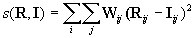(A1)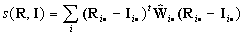(A2)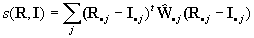(A3)

Where W = {Wij} is the weighting matrix and Ŵj• and Ŵ•j are diagonal matrices with elements determined by the ith row and the jth column of W respectively. All vectors are column vectors.

While (A1) express the closeness between the resolution matrix and the ideal resolution matrix (I) element by element, (A2) and (A3) express the closeness of the rows and columns of the resolution matrix to the ideal rows and ideal columns respectively. Thus optimizing any of these expressions with respect to the inverse G, produce the same results. While (A2) produce very simple estimators for each row of the inverse separately (as we presented in , the same estimators can be derived from (A3) just considering lengthy algebraic transformations related with the vectorization of a product of matrices. Thus, optimization of the rows, i.e., the resolution kernels (using A2) is explicit equivalent to the optimization of the columns, i.e., the spread functions (using A3).

Appendix II: Solution of an underdetermined system of linear equations subject to underdetermined homogeneous linear constraints.

One solution of the problem: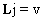s.t.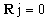is: (A1)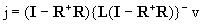(A5)

Based on the properties of the generalized inverses, is not difficult to see that (A5) fulfill both equations in (A4).

References

 Pascual-Marqui, R.D. "Review of methods for solving the EEG inverse problem". International Journal of Bioelectromagnetism. No. 1, Vol.1, 1999.

 Grave de Peralta Menendez R and Gonzalez Andino SL. "A critical analysis of linear inverse solutions". IEEE Trans. Biomed. Engn. Vol 4: 440-48. 1998.

 Grave de Peralta Menendez R, Hauk O, Gonzalez Andino, S, Vogt H and Michel. CM: "Linear inverse solutions with optimal resolution kernels applied to the electromagnetic tomography." Human Brain Mapping, Vol 5: 454-67. 1997.

 Grave de Peralta Menende R., Gonzalez Andino SL. "Distributed source models: Standard solutions and new developments". In: Uhl C, ed. Analysis of Neurophysiological Brain Functioning. Heidelberg: Springer Verlag. 1998.

 Grave de Peralta Menendez R., Gonzalez Andino SL and Lütkenhönner B. "Figures of merit to compare linear distributed inverse solutions". Brain Topography. Vol. 9. No. 2:117-124. 1996

 Pascual-Marqui, R.D. "Reply to comments by Hämäläinen, Ilmoniemi and Nunez. In ISBET Newsletter No. 6, December 1995. Ed: W. Skrandiws. 16-28.

 Grave de Peralta Menendez R, Gonzalez Andino SL, (1998c). Basic limitations of linear inverse solutions: A case study. Proceedings of the 20th annual international conference of the Engineering and Biology Society (EMBS).

 Grave de Peralta Menendez R and Gonzalez Andino, S,: "Backus and Gilbert method for vector fields." Human Brain Mapping, Vol 7: 161-165. 1999.

 Pascual Marqui, RD and Michel, CM (1994) LORETA: New Authentic 3D functional images of the brain. In: ISBET Newsletter No. 5, November 1994. Ed: W. Skrandies. 4-8.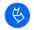Official journal of the International Society for Bioelectromagnetism